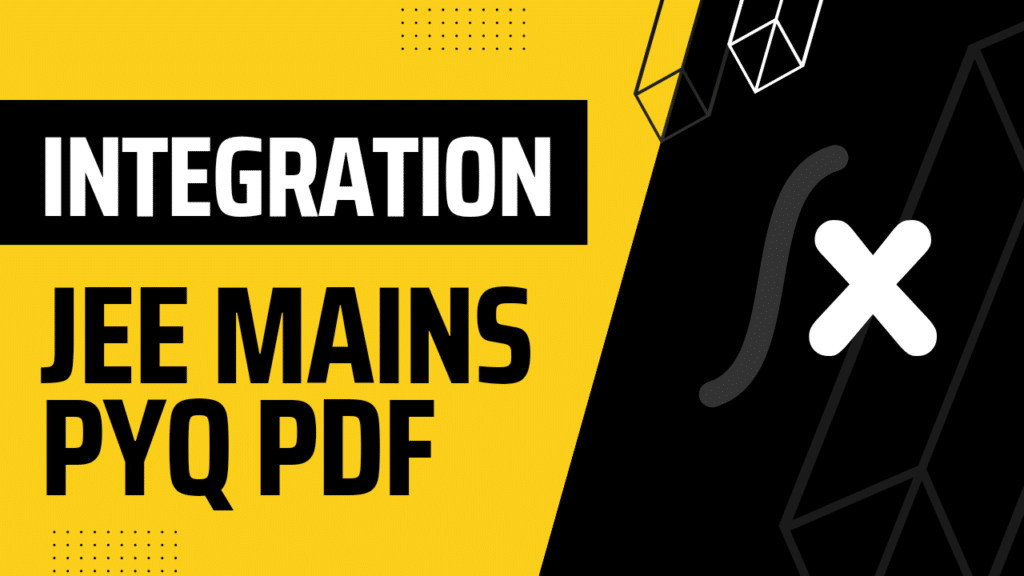Hey Students, read this article and you can download the Integration JEE Mains PYQ PDF. Integration is chapter 7 of class 12 Mathematics and an important chapter for JEE Exams. In this article, you’ll find the top 25 Integration JEE Mains PYQ PDFs. You can get a PDF of the question paper. These questions are taken from the previous year’s JEE Mains Paper and are important to you. You can follow our blog if you want to save notes, mock papers, and previous year’s questions of JEE, Neet, and other competitive exams.

## Is Integration an important chapter for JEE Mains Exams?

The answer is yes, the Integration is an important topic of JEE Mains Exams. You can see the jee mains previous year’s questions, you’ll find that every year there is something from this chapter Integration. So, you have to cover the topics of this chapter too, if you are preparing for the JEE Mains Exam. You will find a link below for “Integration JEE Mains PYQ PDF”

## Chapter-wise Syllabus of Mathematics for JEE Exam

### Topics of Integration For JEE Mains

A. Indefinite Integral

1. Fundamentals of indefinite integral

2. Methods of integration

3. Some special integrals

4. Integration by part

5. S Integration using partial fractions

6. Indirect and derived substitutions

7. Euler’s substitution, reduction formula and integration using differentiation

B. Definite Integral

1. Integration basics, Geometrical interpretation of definite integral, Evaluation of definite integrals by substitution

2. Properties of definite integral

3. Applications of periodic functions and Newton -Leibnitz’s Formula

4. Integration as limit of a sum, Application of inequality, gamma function, beta function and Walli’s Formula

## JEE Previous Year Mathematics Questions PDF

In this blog, you’ll find chapter-wise jee main previous year mathematics question paper pdf. You can simply save a PDF file of the previous year’s question by clicking on the three dots present in Adobe PDF Viewer.

Here is the pdf file of Integration JEE Mains PYQ PDF. You can view pdf files as well as you can simply download the file.I hope so, you like the article “Integration JEE Mains PYQ PDF”. You can find chapter-wise questions for JEE Mains. Download the Integration JEE Mains PYQ PDF by tapping on the three dots(…) mentioned.

#### Previous Year JEE Mains Questions:-

Chemistry Previous Year JEE Mains Questions

Presented By Chavinesh Mukund